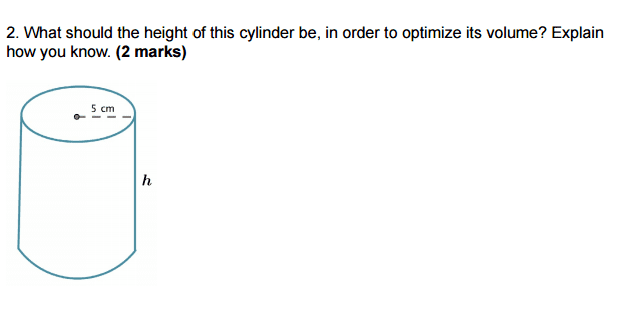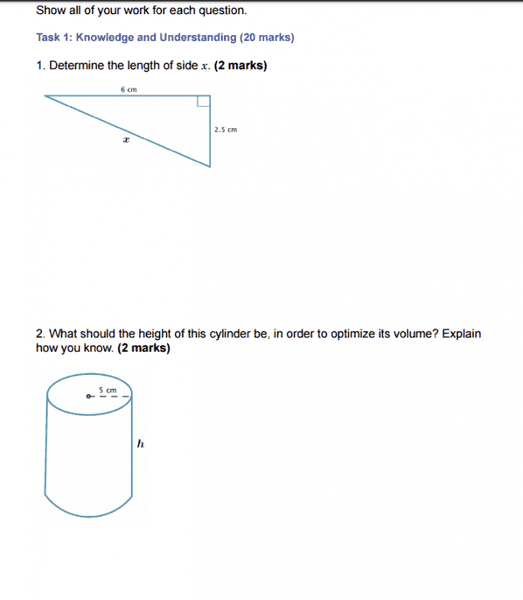# Finding height and volume of a cylinder

• Kirito123

## Homework Statement## Homework Equations

Volume for cylinder = Pi (r)2 h

Optimise : 1. make the best or most effective use of (a situation, opportunity, or resource).
2. rearrange or rewrite (data, software, etc.) to improve efficiency of retrieval or processing.

## The Attempt at a Solution

So I know that the Radius is 5, which means it has a diameter of 10. What i don't understand is how can you find the height without knowing the volume??

If I get the volume I can apply it to this new formula to find the height: H = v / pi(r)2.
I may have misunderstood the question or the meaning of optimise, which is my fault but if you do manage to help me out then THANKS ALOT.

It might be a really simple question but i think i misunderstood what they want or something.

## Homework Statement

View attachment 100625

## Homework Equations

Volume for cylinder = Pi (r)2 h

Optimise : 1. make the best or most effective use of (a situation, opportunity, or resource).
2. rearrange or rewrite (data, software, etc.) to improve efficiency of retrieval or processing.

## The Attempt at a Solution

So I know that the Radius is 5, which means it has a diameter of 10. What i don't understand is how can you find the height without knowing the volume??

If I get the volume I can apply it to this new formula to find the height: H = v / pi(r)2.
I may have misunderstood the question or the meaning of optimise, which is my fault but if you do manage to help me out then THANKS ALOT.
Is this the exact wording of the problem? For the problem you posted, I'm interpreting "optimize the volume" to mean "make the volume as large as possible."

With the radius as shown in the drawing, you can make the volume as large as you want merely by increasing h. IOW, for a given value of h, you can double the volume of the cylinder just by doubling the value of h.

Unless there is some other information that you didn't show, I don't see any way of answering the question in this problem.

Is this the exact wording of the problem? For the problem you posted, I'm interpreting "optimize the volume" to mean "make the volume as large as possible."

Yes this is the exact wording, and that's also why i don't understand the question. There should be more information but that's all they gave.

The best advice I can give is for you to ask your instructor to explain what is meant by "optimize the volume" in this question..

Ok then well thanks a lot anyway.The question you posted is #2. What is question #1? It might have some context that pertains to question 2.

question 1 is talking about Pythagorean theorem, which has nothing to do with this. there is no relation between them or else i would have posted. I just sent my instructor a message.I agree the wording isn't clear. My guess at the intent is to find the height that gives the most volume per unit area of wall. This would be a problem with a non-trivial answer and a real world application (how do I use the least steel and get the most capacity in my drum?).

well i sent my teacher a message waiting for a reply, at least now i know the question didn't make sense to only me :)